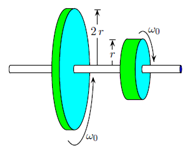# Problem: Two disks of identical mass but different radii (r and 2 r) are spinning on frictionless bearings at the same angular speed ω0 but in opposite directions. The two disks are brought slowly together. The resulting frictional force between the surfaces eventually brings them to a common angular velocity. What is the magnitude of that final angular velocity in terms of ω0? 1. ωf = 2/3 ω0 2. ωf = 1/5 ω0 3. ωf = 1/3 ω0 4. ωf = 1/2 ω0 5. ωf = 4/5 ω0 6. ωf = 3/5 ω0 7. ωf = 3/4 ω0 8. ωf = 2/5 ω0 9. ωf = 1/4 ω0

###### FREE Expert Solution
80% (251 ratings)
###### Problem Details

Two disks of identical mass but different radii (r and 2 r) are spinning on frictionless bearings at the same angular speed ω0 but in opposite directions. The two disks are brought slowly together. The resulting frictional force between the surfaces eventually brings them to a common angular velocity. What is the magnitude of that final angular velocity in terms of ω0?

1. ωf = 2/3 ω0

2. ωf = 1/5 ω0

3. ωf = 1/3 ω0

4. ωf = 1/2 ω0

5. ωf = 4/5 ω0

6. ωf = 3/5 ω0

7. ωf = 3/4 ω0

8. ωf = 2/5 ω0

9. ωf = 1/4 ω0